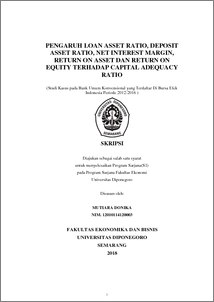# PENGARUH LOAN ASSET RATIO, DEPOSIT ASSET RATIO, NET INTEREST MARGIN, RETURN ON ASSET DAN RETURN ON EQUITY TERHADAP CAPITAL ADEQUACY RATIO (Studi Kasus pada Bank Umum Konvensional yang Terdaftar Di Bursa Efek Indonesia Periode 2012-2016 )

DONIKA , Mutiara and INDRIANI , Astiwi (2018) PENGARUH LOAN ASSET RATIO, DEPOSIT ASSET RATIO, NET INTEREST MARGIN, RETURN ON ASSET DAN RETURN ON EQUITY TERHADAP CAPITAL ADEQUACY RATIO (Studi Kasus pada Bank Umum Konvensional yang Terdaftar Di Bursa Efek Indonesia Periode 2012-2016 ). Undergraduate thesis, Fakultas Ekonomika dan Bisnis.Preview
PDF - Published Version
1627Kb

## Abstract

This research was conducted to examine the influence of the Loan Asset Ratio / LAR, Deposit Asset Ratio / DAR, Net Interest Margin / NIM, Return On Asset / ROA and Return On Equity / ROE toward Capital Adequacy Ratio. The study population consisted of 18 Conventional Commercial Banks listed in Indonesia Stock Exchange (IDX) period 2012 -2016. The sample selection using purposive sampling method, with criteria have complete financial report and ROA and ROE positive. So that obtained a sample of 14 banks with 70 observation data.Data analysis used multiple regression test equipment that included classical assumption test consisting of normality test, multicolonierity test, autocorrelation test and heterokedastisity test. While hypothesis testing is done by F test and T test. The results of this test indicate that Return On Asset / ROA has a significant positive effect on Capital Adequacy Ratio / CAR. While Return On Equity / ROE have significant negative effect to Capital Adequacy Ratio / CAR. Meanwhile, Loan Asset Ratio / LAR and Net Interest Margin / NIM variables have no significant negative effect on Capital Adequacy Ratio / CAR. Deposit Asset Ratio / DAR has an insignificant positive effect on CAR. Adjusted R Square value of 0.257. This indicates the amount of influence given by Loan Asset Ratio / LAR, Deposit Asset Ratio / DAR, Net Interest Margin / NIM, Return On Asset / ROA and Return On Equity / ROE to CAR change is 25.7%. while the remaining 74.7% can be explained by other variables outside the model.

Item Type: Thesis (Undergraduate) Capital Adequacy Ratio / CAR, Loan Asset Ratio / LAR, Deposit Asset Ratio / DAR, Net Interest Margin / NIM, Return On Asset / ROA dan Return On Equity / ROE. Capital Adequacy Ratio / CAR, Loan Asset Ratio / LAR, Deposit Asset Ratio / DAR, Net Interest Margin / NIM, Return On Asset / ROA dan Return On Equity / ROE. H Social Sciences > H Social Sciences (General) Faculty of Economics and Business > Department of Management 62024 Mr. Perpustakaan Fakultas Ekonomi 23 Apr 2018 09:44 23 Apr 2018 09:44

Repository Staff Only: item control page